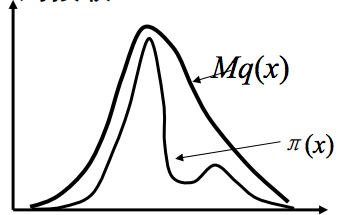# 随机模拟的基本思想和常用抽样方法

$$\inf _a^b f(x)*g(x)/g(x) dx = \inf _a^b (1/g(x)) *f(x)*g(x) dx$$

（pku，sewm，shinning）

2.0 直接抽样法

2.1 接受-拒绝抽样（Acceptance-Rejection sampling)

1. 对于g(x)进行采样得到一个样本xi, xi ~ g(x);

2. 对于均匀分布采样 ui ~ U(a,b);

3. 如果ui<= f(x)/[M*g(x)], 那么认为xi是有效的样本；否则舍弃该样本； （# 这个步骤充分体现了这个方法的名字：接受-拒绝）

4. 反复重复步骤1~3，直到所需样本达到要求为止。(说明：这是从其他地方弄来的图，不是自己画的，符号有些和文中不一致，其中\pi(x) 就是文中的f(x)，q(x)就是文中的g(x)  )

2.2 重要性抽样(Importance sampling)

$\inf f(x) dx = \inf f(x)*(1/g(x))*g(x) dx$, 如果g(x)是一个概率分布，从g(x)中抽取N个样本，上述的式子就约等于$(1/N)* \sum f(xi)*(1/g(xi))$。这相当于给每个样本赋予了一个权重，g(xi)大意味着概率大，那么N里面含有这样的样本xi就多，即这些样本的权重大，所以称为重要性抽样。

1. 选择一个容易抽样的分布g(x), 从g(x)中抽取N个样本；
2. 计算(1/N)* \sum f(xi)*(1/g(xi))，从而得到近似解。

（pku，sewm，shinning）

2.3  MCMC抽样方法

A).  Metropolis-Hasting算法

1. 给定一个起始样本$x_0$和一个建议分布q(y|x)；

2. 对于第i个样本xi，通过q(y|xi)得到一个建议样本y；计算比率$\alpha(xi, y)= min{1, q(xi|y)*\pi(y)/[q(y|xi)*\pi(xi)]}$;

3. 抽取一个均匀分布样本ui ~ U(0,1)，如果ui <= \alpha(xi,y)，则x_{i+1} = y；否则x_{i+1} = xi；

4. 重复步骤2~3，直到抽取到想要的样本数量为止。

B).  Gibbs采样算法

Gibbs算法，很简单，就是用条件分布的抽样来替代全概率分布的抽样。例如，X={x1,x2,...xn}满足分布p(X)，如何对p(X)进行抽样呢？如果我们知道它的条件分布p(x1|X_{-1}),...,p(xi|X_{-i}),....，其中X_{-i}表示除了xi之外X的所有变量。如果这些条件分布都是很容易抽样的，那么我们就可以通过对条件分布的抽样来对全概率分布p(X)进行抽样。

Gibbs采样算法的步骤：

1. 给定一个初始样本X0={x10,x20,...,xn0}

2.已知一个样本Xi={x1i,x2i,...,xni}，对于x1_{i+1}进行抽样，x1_{i+1} ~ p(x1|Xi_{-1})

3. 对于x2_{i+1}进行抽样，x2_{i+1} ~ p(x2|x1_{i+1}, x2i,...xni)

................................................................

4.对于xn_{i+1}进行抽样，xn_{i+1} ~ p(xn|x1_{i+1}, x2_{i+1},...x_{n-1}_{i+1})

5.步骤2~4可以得到X的一个样本，然后重复步骤2~4可以不断地得到X的样本。

• 本文由 发表
• 转载请务必保留本文链接：https://www.plob.org/article/5160.html